# NCERT Solutions for Class 10 Maths Chapter 13 - Surface Areas and Volumes

Share

NCERT Mathematics Solutions for Class 10th Chapter 13- Surface Areas and Volumes solved by an experienced team of Goprep to aid students in understanding the concepts of the topics in an efficient way. Crafted by a qualified subject mentor, these Solutions are prepared in accordance with the latest official curriculum in order to help students in preparing for their board examinations.

Surface Areas and Volumes are one of the important chapters of Class 10 as this deals with the important concepts like Area and Volume, Basic elements of Volume, Combinations of Solids, Surface Area of a Combination of Solids, Conversion of Solid from one shape to another and Frustum of a Cone, etc. Providing step-by-step answers to the questions, you can perform better and score more marks in the exam by using these NCERT Solutions.

## NCERT Solutions for Class 10 Maths Chapter 13 - Surface Areas and Volumes

Exercise 13.1
• Exercise 13.1
• Exercise 13.2
• Exercise 13.3
• Exercise 13.4
• Exercise 13.5
Class 10th|NCERT - MathematicsChapter 13 - Surface Areas and Volumes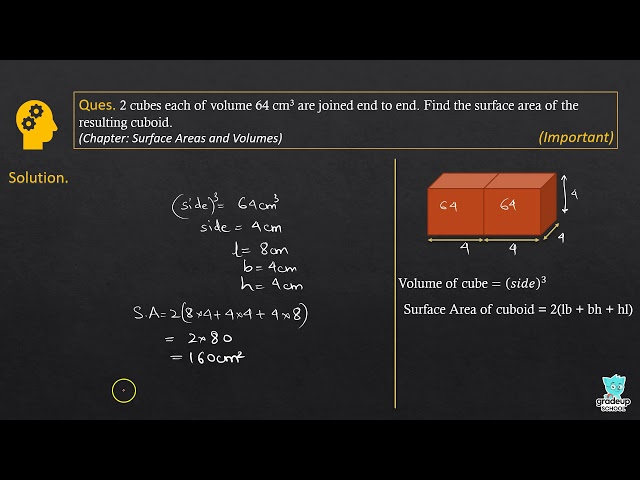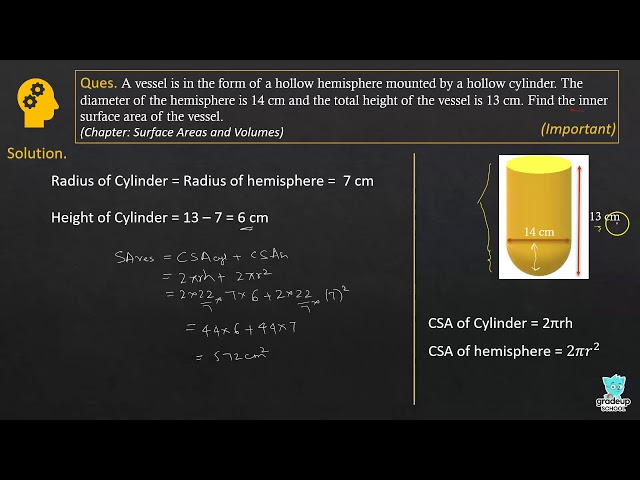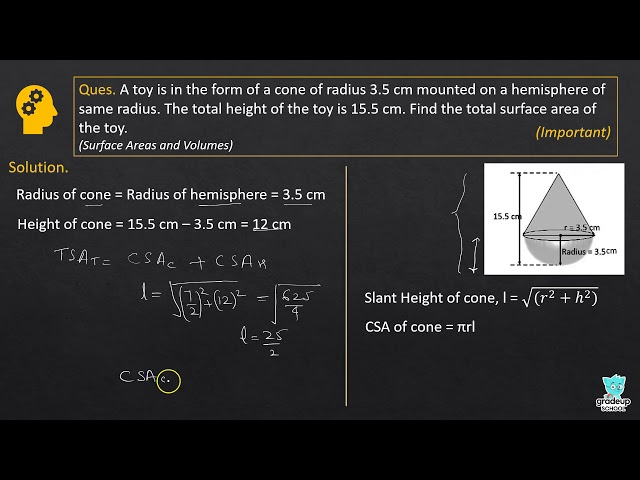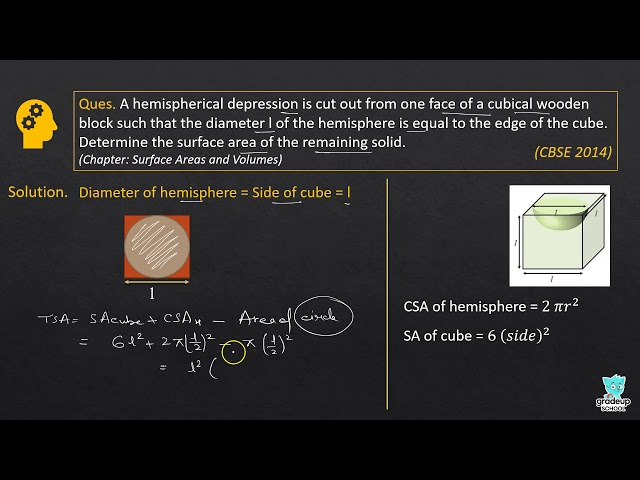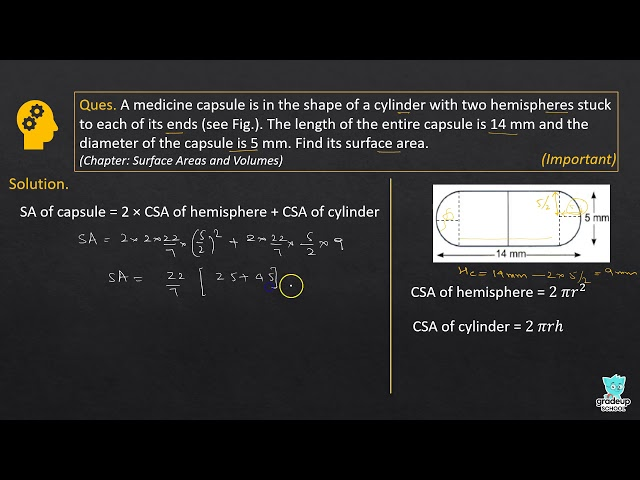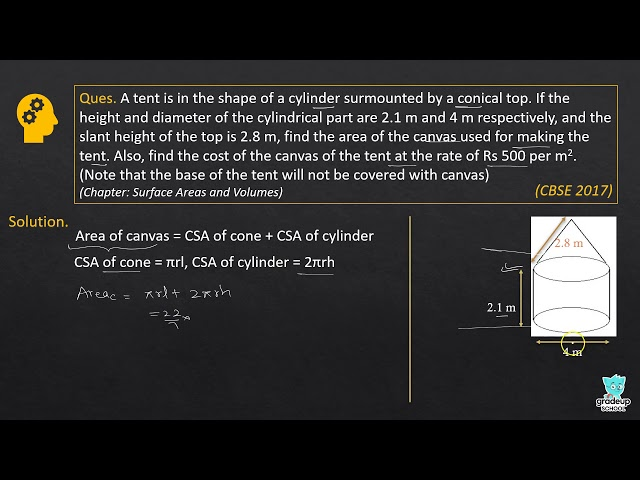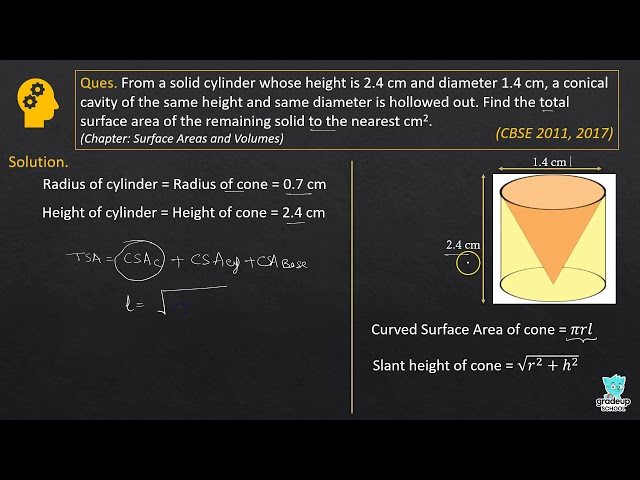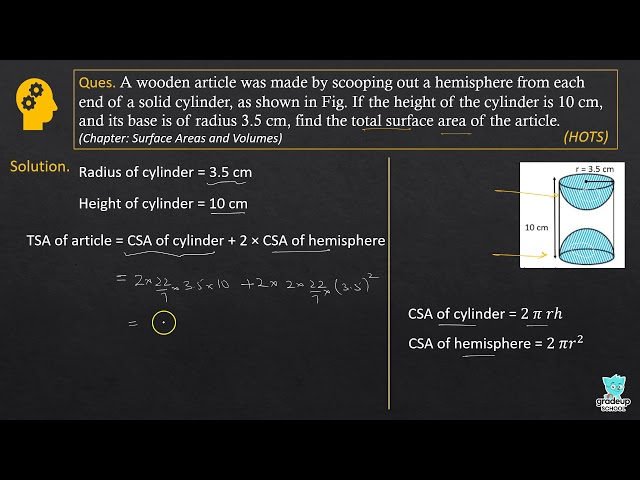NCERT - MathematicsClass 10th , NCERT
 Chapter 1 - Real Numbers Chapter 2 - Polynomials Chapter 3 - Pair of Linear Equations in Two Variables Chapter 4 - Quadratic Equations Chapter 5 - Arithmetic Progressions Chapter 6 - Triangles Chapter 7 - Coordinate Geometry Chapter 8 - Introduction to Trigonometry Chapter 9 - Some Applications of Trigonometry Chapter 10 - Circles Chapter 11 - Constructions Chapter 12 - Areas Related to Circles Chapter 13 - Surface Areas and Volumes Chapter 14 - Statistics Chapter 15 - Probability